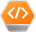• python二进制转十进制
千次阅读
2021-12-15 14:23:52
十进制转换为8位二进制 ：format(int(26),'08b')

十进制转换为四位二进制 ：format(int(4),'04b')

二进制转换为十进制 ：int("00011000",2)开发语言 后端
更多相关内容
• python十进制转二进制 python十进制转二进制使用 bin() 函数。 bin() 返回一个整数 int 或者长整数 long int 的二进制表示。 下面是使用示例： >>>bin(10) '0b1010' >>> bin(20) '0b10100' 补充：十进制转8进制和...
• 绒绒小梧桐2020-02-24 10:13:41二进制转十进制,十进制转二进制的算法十进制转二进制:用2辗转相除至结果为1将余数和最后的1从下向上倒序写 就是结果例如302302/2 = 151 余0151/2 = 75 余175/2 = 37 余137/2 = 18 余...

绒绒小梧桐2020-02-24 10:13:41

二进制转十进制,十进制转二进制的算法

十进制转二进制:

用2辗转相除至结果为1

将余数和最后的1从下向上倒序写 就是结果

例如302

302/2 = 151 余0

151/2 = 75 余1

75/2 = 37 余1

37/2 = 18 余1

18/2 = 9 余0

9/2 = 4 余1

4/2 = 2 余0

2/2 = 1 余0

故二进制为100101110

二进制转十进制

从最后一位开始算,依次列为第0、1、2...位

第n位的数(0或1)乘以2的n次方

得到的结果相加就是答案

例如:01101011.转十进制:

第0位:1乘2的0次方=1

1乘2的1次方=2

0乘2的2次方=0

1乘2的3次方=8

0乘2的4次方=0

1乘2的5次方=32

1乘2的6次方=64

0乘2的7次方=0

然后:1+2+0

+8+0+32+64+0=107.

二进制01101011=十进制107.

一、二进制数转换成十进制数

由二进制数转换成十进制数的基本做法是,把二进制数首先写成加权系数展开式,然后按十进制加法规则求和。这种做法称为"按权相加"法。

二、十进制数转换为二进制数

十进制数转换为二进制数时,由于整数和小数的转换方法不同,所以先将十进制数的整数部分和小数部分分别转换后,再加以合并。

1. 十进制整数转换为二进制整数

十进制整数转换为二进制整数采用"除2取余,逆序排列"法。具体做法是:用2去除十进制整数,可以得到一个商和余数;再用2去除商,又会得到一个商和余数,如此进行,直到商为零时为止,然后把先得到的余数作为二进制数的低位有效位,后得到的余数作为二进制数的高位有效位,依次排列起来。

2.十进制小数转换为二进制小数

十进制小数转换成二进制小数采用"乘2取整,顺序排列"法。具体做法是:用2乘十进制小数,可以得到积,将积的整数部分取出,再用2乘余下的小数部分,又得到一个积,再将积的整数部分取出,如此进行,直到积中的小数部分为零,或者达到所要求的精度为止。

然后把取出的整数部分按顺序排列起来,先取的整数作为二进制小数的高位有效位,后取的整数作为低位有效位。

回答者:HackerKinsn - 试用期 一级 2-24 13:31

1.二进制与十进制的转换

(1)二进制转十进制

方法:"按权展开求和"

例:

(1011.01)2 =(1×23+0×22+1×21+1×20+0×2-1+1×2-2)10

=(8+0+2+1+0+0.25)10

=(11.25)10

(2)十进制转二进制

· 十进制整数转二进制数:"除以2取余,逆序输出"

例: (89)10=(1011001)2

2 89

2 44 …… 1

2 22 …… 0

2 11 …… 0

2 5 …… 1

2 2 …… 1

2 1 …… 0

0 …… 1

· 十进制小数转二进制数:"乘以2取整,顺序输出"

例:

(0.625)10= (0.101)2

0.625

X 2

1.25

X 2

0.5

X 2

1.0

2.八进制与二进制的转换

例:将八进制的37.416转换成二进制数:

37 . 4 1 6

011 111 .100 001 110

即:(37.416)8 =(11111.10000111)2

例:将二进制的10110.0011 转换成八进制:

0 1 0 1 1 0 . 0 0 1 1 0 0

2 6 . 1 4

即:(10110.011)2 =(26.14)8

3.十六进制与二进制的转换

例:将十六进制数5DF.9 转换成二进制:

5 D F . 9

0101 1101 1111.1001

即:(5DF.9)16 =(10111011111.1001)2

例:将二进制数1100001.111 转换成十六进制:

0110 0001 . 1110

6 1 . E

即:(1100001.111)2 =(61.E)16

展开全文• 学习python基础过程中的实例及问题，主要是python学习手册第五版内容已经遇到的其他有趣的实例，在此分享。如有侵权，联系删除
• 主要介绍了python十进制二进制的转换方法(含浮点数)，小编觉得挺不错的，现在分享给大家，也给大家做个参考。一起跟随小编过来看看吧
• 二进制数从右向左每位数乘以2的次方（从0开始，从右向左依次+1），然后相加求和即可如：0101十进制为：1*20+0*21+1*22+0*23=1+0+4+0=5算法实现：#coding=utf-8b=raw_input("请输入一个二进制数：".decode("utf-8...

二进制数转换成十进制数：二进制数从右向左每位数乘以2的次方（从0开始，从右向左依次+1），然后相加求和即可

如：0101转成十进制为：1*20+0*21+1*22+0*23=1+0+4+0=5

算法实现：

#coding=utf-8

b=raw_input("请输入一个二进制数：".decode("utf-8").encode("gbk"))

sum=0

for i in range(len(b)):

sum+=int(b[len(b)-1-i])*pow(2,i)

print u"转换成十进制数是：",sum

-------------------------------------------

十进制数转换成二进制数：十进制数除2取余，商继续除2取余，直到商为0，所有余数逆顺即可

如：12转换成二进制是：1100

12/2=6 ------ 0 第4位

6/2=3 ------ 0 第3位

3/2=1 ------ 1 第2位

1/2=0 ------ 1 第1位

算法实现：

#coding=utf-8

n=int(raw_input(u"请输入一个正整数：".encode("gbk")))

res=[]

res.append(str(n%2))

while n/2!=0:

n=n/2

res.append(str(n%2))

res.reverse()

print u"转化为二进制是：","".join(res)

展开全文• 本文实例讲述了Python实现的十进制小数与二进制小数相互转换功能。分享给大家供大家参考，具体如下： 十进制小数 ⇒ 二进制小数 乘2取整 对十进制小数乘2得到的整数部分和小数部分， 整数部分即是相应的二进制数码，...
• 下面我们开讲原理，举个十进制整数转换为二进制整数的例子，假设十进制整数A化得的二进制数为edcba 的形式，那么用上面的方法按权展开， 得 A=a(2^0)+b(2^1)+c(2^2)+d(2^3)+e(2^4) （后面的和不正是化十进制的过程...

如：255=（11111111）B255/2=127=====余1127/2=63======余163/2=31=======余131/2=15=======余115/2=7========余17/2=3=========余13/2=1=========余11/2=0=========余1789=1100010101(B)789/2=394余1 第10位394/2=197余0 第9位197/2=98余1 第8位98/2=49余0 第7位49/2=24余1 第6位24/2=12余0 第5位12/2=6余0 第4位6/2=3余0 第3位3/2=1余1 第2位1/2=0余1 第1位

原理：

众所周知，二进制的基数为2，我们十进制化二进制时所除的2就是它的基数。谈到它的原理，就不得不说说关于位权的概念。某进制计数制中各位数字符号所表示的数值表示该数字符号值乘以一个与数字符号有关的常数，该常数称为 “位权 ” 。位权的大小是以基数为底，数字符号所处的位置的序号为指数的整数次幂。十进制数的百位、十位、个位、十分位的权分别是10的2次方、10的1次方、10的0次方，10的-1次方。二进制数就是2的n次幂。

按权展开求和正是非十进制化十进制的方法。

下面我们开讲原理，举个十进制整数转换为二进制整数的例子，假设十进制整数A化得的二进制数为edcba 的形式，那么用上面的方法按权展开， 得

A=a(2^0)+b(2^1)+c(2^2)+d(2^3)+e(2^4) （后面的和不正是化十进制的过程吗）

假设该数未转化为二进制,除以基数2得

A/2=a(2^0)/2+b(2^1)/2+c(2^2)/2+d(2^3)/2+e(2^4)/2注意：a除不开二，余下了！其他的绝对能除开，因为他们都包含2，而a乘的是1，他本身绝对不包含因数2，只能余下。

商得：

b(2^0)+c(2^1)+d(2^2)+e(2^3)，再除以基数2余下了b，以此类推。

当这个数不能再被2除时，先余掉的a位数在原数低，而后来的余数数位高，所以要把所有的余数反过来写。正好是edcba

展开全文• 1、十进制二进制之间的转换(1)、十进制转换为二进制，分为整数部分和小数部分整数部分方法：除2取余法，即每次将整数部分除以2，余数为该位权上的数，而商继续除以2，余数又为上一个位权上的数。这个步骤一直...
• 十进制转换成二进制 十进制转换成二进制，采用的是“除以2求余数”的计算方法，如下所示： “除以2”的过程，得到的余数是从低到高的次序，而需要的带的输出是从高位到低位（35对应的二进制是100011），因此需要一...
• 其实这是非常简单的，这个就像小学学习数学乘法口诀意义，只要记住转换口诀即可轻松应用，一起来看下具体的操作内容吧~一、python进制转换dec（十进制）—> bin（二进制）dec（十进制）—> oct（八进制）dec（十进制...
• #二进制转化成十六进制print("请输入一个二进制数\n")SUM1=[]SUM2=[]A=0B=0int1=[]#整数部分存放处float1=[]#小数部分存放处a1=int(input("请输入二进制的整数部分"))print("a1=",a1)#整数部分a2=input("请输入...
• python input函数进制转换 input()函数-控制输入的进制数 格式化符号 ... s=int(input(),2) #输入二进制并将其转换为十进制 通过格式化符号进行转化 这里用输入一个十六进制的数为例子 n=int(input(),
• 这篇文章主要介绍了使用Python内置函数：bin()、oct()、int()、hex()可实现进制转换的一些用法,需要的朋友可以参考下 使用Python内置函数：bin()、oct()、int()、hex()可实现进制转换。 先看Python官方文档中对这几...
• 以及Python十进制二进制相互之间的转换 def zbinary(num): #判断是否为整数 if num == int(num): integer = '{:b}'.format(int(num)) #将十进制整数转换为二进制数 return num else: integer_part = int...开发语言 后端
• 主要介绍了使用Python内置函数：bin()、oct()、int()、hex()可实现进制转换的一些用法,需要的朋友可以参考下
• 主要介绍了Python中不同进制互相转换,本文讲解了二进制、八进制、十进制和十六进制的相与转换实现代码,需要的朋友可以参考下
• https://www.xin3721.com/eschool/pythonxin3721/使用内置函数实现进制转换实现比较简单...二进制转十进制使用函数：int()实例：123#结果是4int('100',2)注意：上述一定不要忘记加引号，因为二进制是一个字符串如果...
• 你好，我是悦创。 接下来来编写 Python 中的进制转换，我们来一一编写。...二进制 十进制 In : int("10100111110", 2) Out: 1342 八进制 十进制 In : int("17", 8) Out: 15 其实可以看到，不开发语言 后端
• 写在前面： 最近在学习数据分析方向的课程，开始系统地学习Python，越来越对“人生苦短，故我用python”这句话有感触了，Python在某些方面确实有其过其他语言之处。...此外用Python进制转换的优势有至少
• 十进制转换为8位二进制 ：format(int(26),'08b') 十进制转换为四位二进制 ：format(int(4),'04b') 二进制转换为十进制 ：int("00011000",2)
• Python：运行时警告：溢出遇到平方，加，乘，减问I'm beginning with Python so I might be asking a not so subtle question, but after quite a lot of research I couldn't resolve this error. I'm actually ...
• # 十进制转化二进制 """ 知识点： 1、数据类型转换 2、赋值运算符，注意：一定要先定义变量 3、逻辑运算符，or and not 4、Wile/for循环 5、算术运算符，整除'//',求余'%' 6、字符串索引 """ while True: ...
• Im doing a colledge assignment which is have us create a python program to convert binary to decimal without using the bin() function or list(). I'm plan to have each 1's and 0's stored in a function....
• 好的，首先在这里发帖，如果问题不可行，请原谅并忽略背景：我是计算机科学160。...提示是读取只包含1和0的整数，逐位处理二进制数并报告十进制等效值。现在，我从一个同学那里得到了一些提示，这至少...
• 文章目录@[toc]1、内置函数转换bin,oct,int,hex1.1 10进制到2，8，16进制字符串1.2 2，8，16进制字符串到10进制整数1.3 各进制互 ...十进制8转换为二进制（’0b’是二进制前缀） oct(15) ‘0o17’...